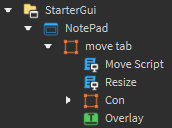# Gui Resizeing Not Working Right

Im making a game where your on a computer and i need help fixing this problem im having with the app resizing i want it to look like this:

and while making it it came out like this:

the part thats not resizing is the part for moving the gui around i want it to only move around when you drag the tab at the top

the plus in the bottom right is the button you use to resize the gui

the resizeing is also very snappy when i want it smooth

The Size Script

``````local Gui = script.Parent
local Con = Gui.Con
local Over = Gui.Overlay

Con.Drag.MouseButton1Down:connect(function()
Over.Visible = true
local Move = Over.MouseMoved:connect(function(X,Y)
local x = X-Con.AbsolutePosition.X-(Con.Drag.Size.X.Offset/2)
local y = math.floor(((Y-(Con.Drag.Size.Y.Offset/2))/20)+0.5)*20
if x < 400 then x = 400 end
if y < 100 then y = 100 end
Con.Size = UDim2.new(0,x,0,y)
local Z = 0
end)
local Up
local Leave
Up = Over.MouseButton1Up:connect(function()
Move:disconnect()
Up:disconnect()
Leave:disconnect()
Over.Visible = false
end)
Leave = Over.MouseLeave:connect(function()
Move:disconnect()
Up:disconnect()
Leave:disconnect()
Over.Visible = false
end)
end)
``````

Moving Script

``````local dragger = {};
local resizer = {};

do
local mouse = game:GetService("Players").LocalPlayer:GetMouse();
local inputService = game:GetService('UserInputService');
local heartbeat = game:GetService("RunService").Heartbeat;
-- // credits to Ririchi / Inori for this cute drag function :)
function dragger.new(frame)
local s, event = pcall(function()
return frame.MouseEnter
end)

if s then
frame.Active = true;

event:connect(function()
local input = frame.InputBegan:connect(function(key)
if key.UserInputType == Enum.UserInputType.MouseButton1 then
local objectPosition = Vector2.new(mouse.X - frame.AbsolutePosition.X, mouse.Y - frame.AbsolutePosition.Y);
while heartbeat:wait() and inputService:IsMouseButtonPressed(Enum.UserInputType.MouseButton1) do
frame:TweenPosition(UDim2.new(0, mouse.X - objectPosition.X + (frame.Size.X.Offset * frame.AnchorPoint.X), 0, mouse.Y - objectPosition.Y + (frame.Size.Y.Offset * frame.AnchorPoint.Y)), 'Out', 'Quad', 0.1, true);
end
end
end)

local leave;
leave = frame.MouseLeave:connect(function()
input:disconnect();
leave:disconnect();
end)
end)
end
end

function resizer.new(p, s)
p:GetPropertyChangedSignal('AbsoluteSize'):connect(function()
s.Size = UDim2.new(s.Size.X.Scale, s.Size.X.Offset, s.Size.Y.Scale, p.AbsoluteSize.Y);
end)
end
end
script.Parent.Active = true
script.Parent.Draggable = true
``````

This is where there locatedand if you want the .rblx here it is
resizeable and moveable gui.rbxl (44.2 KB)

this is the best i can do so if you have any more questions on my problems or you have a solution reply

I haven’t really read through your code yet, but it looks like the top-bar isn’t changing size together with the rest of the window. In your GUI picture I am guessing that `con` is the thing that is being resized, and `move tab` is what isn’t being resized.

If you in the code handling resizing make it change the X-size of `move tab` then the `X-size` of `con` will automatically change as well (if its X-size is: `Scale = 1`)

this is what happens when i change the x size with Con

as you can see when i make gui change x with con (Gui is the moving tab)
it breaks and doesnt move at all and it doesnt say anything in the output thats wrong

this is the new code

``````local Gui = script.Parent
local Con = Gui.Con
local Over = Gui.Overlay

Con.Drag.MouseButton1Down:connect(function()
Over.Visible = true
local Move = Over.MouseMoved:connect(function(X,Y)
local x = X-Con.AbsolutePosition.X-(Con.Drag.Size.X.Offset/2)
local y = math.floor(((Y-(Con.Drag.Size.Y.Offset/2))/20)+0.5)*20
if x < 400 then x = 400 end
if y < 100 then y = 100 end
Con.Size = UDim2.new(0,x,0,y)
Gui.Size = UDim2.new(0,x)
local Z = 0
end)
local Up
local Leave
Up = Over.MouseButton1Up:connect(function()
Move:disconnect()
Up:disconnect()
Leave:disconnect()
Over.Visible = false
end)
Leave = Over.MouseLeave:connect(function()
Move:disconnect()
Up:disconnect()
Leave:disconnect()
Over.Visible = false
end)
end)
``````

I think the issue is you are changing con’s X as well as the parent’s X. What I meant is that you do something like this:

``````local Gui = script.Parent
local Con = Gui.Con
local Over = Gui.Overlay

-- This is meant to make sure the sizing is correctly made.
--  This should preferably be done in the properties tab. [change X size to (1, 0)]
con.Size = UDim2.new(1, 0, con.Size.Y.Scale, con.Size.Y.Offset)

Con.Drag.MouseButton1Down:connect(function()
Over.Visible = true
local Move = Over.MouseMoved:connect(function(X,Y)
local x = X-Con.AbsolutePosition.X-(Con.Drag.Size.X.Offset/2)
local y = math.floor(((Y-(Con.Drag.Size.Y.Offset/2))/20)+0.5)*20
if x < 400 then x = 400 end
if y < 100 then y = 100 end
Con.Size = UDim2.new(1, 0, 0, y) -- Only change the Y value
-- Gui.Size = UDim2.new(0,x)   <-- You do not assign a Y value here, which will cause an error
Gui.Size = UDim2.new(0, x, Gui.Size.Y.Offset, Gui.Size.Y.Scale) -- Keep original Y values
local Z = 0
end)
local Up
local Leave
Up = Over.MouseButton1Up:connect(function()
Move:disconnect()
Up:disconnect()
Leave:disconnect()
Over.Visible = false
end)
Leave = Over.MouseLeave:connect(function()
Move:disconnect()
Up:disconnect()
Leave:disconnect()
Over.Visible = false
end)
end)
``````

I’m writing this in the devforum editor, so it’s possible I made some mix ups when it comes to names and spelling mistakes, but hopefully you get the picture!